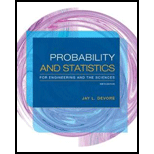# Show that for any Δ &gt; 0, when the population distribution is normal and σ is known, the two-tailed test satisfies β ( μ 0 − Δ) = β ( μ 0 + Δ), so that β ( μ ′) is symmetric about μ 0 .### Probability and Statistics for Eng...

9th Edition
Jay L. Devore
Publisher: Cengage Learning
ISBN: 9781305251809### Probability and Statistics for Eng...

9th Edition
Jay L. Devore
Publisher: Cengage Learning
ISBN: 9781305251809

#### Solutions

Chapter
Section
Chapter 8.2, Problem 27E
Textbook Problem

## Show that for any Δ > 0, when the population distribution is normal and σ is known, the two-tailed test satisfies β(μ0 − Δ) = β(μ0 + Δ), so that β(μ′) is symmetric about μ0.

Expert Solution
To determine

Show that for two tailed test, β(μ0Δ)=β(μ0+Δ).

### Explanation of Solution

Given info:

The population distribution is normal and the population standard deviation is known. The additional information is that if β(μ0Δ)=β(μ0+Δ) then β(μ') will be symmetric about μ0.

Calculation:

From the definition of β, for two tailed test,

β(μ0Δ)=Φ(zα2+Δσn)Φ(zα2+Δσn) (1)

Where Φ() is the cdf of the standard normal distribution.

It is known that Φ(z)=1Φ(z)

Assuming zα2+Δσn as –z, Φ(zα2+Δσn)=1Φ(zα2Δσn) (2)

And assuming zα2+Δσn as –z, Φ(zα2+Δσn)=1Φ(zα2Δσ<

### Want to see the full answer?

Check out a sample textbook solution.See solution

### Want to see this answer and more?

Bartleby provides explanations to thousands of textbook problems written by our experts, many with advanced degrees!

See solution

Find more solutions based on key concepts
Show solutions
Identify the four steps of a hypothesis test as presented in this chapter.

Statistics for The Behavioral Sciences (MindTap Course List)

Let g be the function defined by g(u) = (3u 2)3/2. Find g(1), g(6), g(113), and g(u + 1).

Applied Calculus for the Managerial, Life, and Social Sciences: A Brief Approach

In Exercises 516, evaluate the given quantity. log416

Finite Mathematics and Applied Calculus (MindTap Course List)

Evaluate the integral. 32xx2dx

Calculus (MindTap Course List)

True or False: y′ + xey = ex+y is separable.

Study Guide for Stewart's Single Variable Calculus: Early Transcendentals, 8th

Explain why plagiarism is unethical.

Research Methods for the Behavioral Sciences (MindTap Course List)

Graph each rational function. f(x)=x3+xx24

College Algebra (MindTap Course List)

Unnecessary Medical Care. According to Reader’s Digest, 42% of primary care doctors think their patients receiv...

Modern Business Statistics with Microsoft Office Excel (with XLSTAT Education Edition Printed Access Card) (MindTap Course List)

Solve the system in Problem 7 subject to the initial condition X(0)=(146). 7. X=(111111222)X

A First Course in Differential Equations with Modeling Applications (MindTap Course List)

Locating the Vertex of a Parabola In Exercises 1 through 4, find the vertex of the parabola that is the graph o...

Functions and Change: A Modeling Approach to College Algebra (MindTap Course List)